Have a scribble pad to hand when playing this quiz.

# Algebra Intermediate - Factoring Trinomials [First term is 1]

This Math quiz is called 'Algebra Intermediate - Factoring Trinomials [First term is 1]' and it has been written by teachers to help you if you are studying the subject at middle school. Playing educational quizzes is a fabulous way to learn if you are in the 6th, 7th or 8th grade - aged 11 to 14.

It costs only \$12.50 per month to play this quiz and over 3,500 others that help you with your school work. You can subscribe on the page at Join Us

Do you remember what trinomials are? Trinomials are when you have three terms in a mathematical problem. When you are then asked to “factor” a trinomial, “factoring” tells you that you need to multiply them. However, how do you do that when the problem clearly shows addition?

When factoring trinomials what you are really doing is working backwards. In other words, what two binomial groupings when multiplied together will give you the trinomial problem. Before we get to solving that, you need a quick refresher on the FOIL system. Do you remember that system?

FOIL stands for: first, outer, inner and last. In other words, it is how you multiply two binomial factors. Let’s look at the following example below.

Go straight to Quiz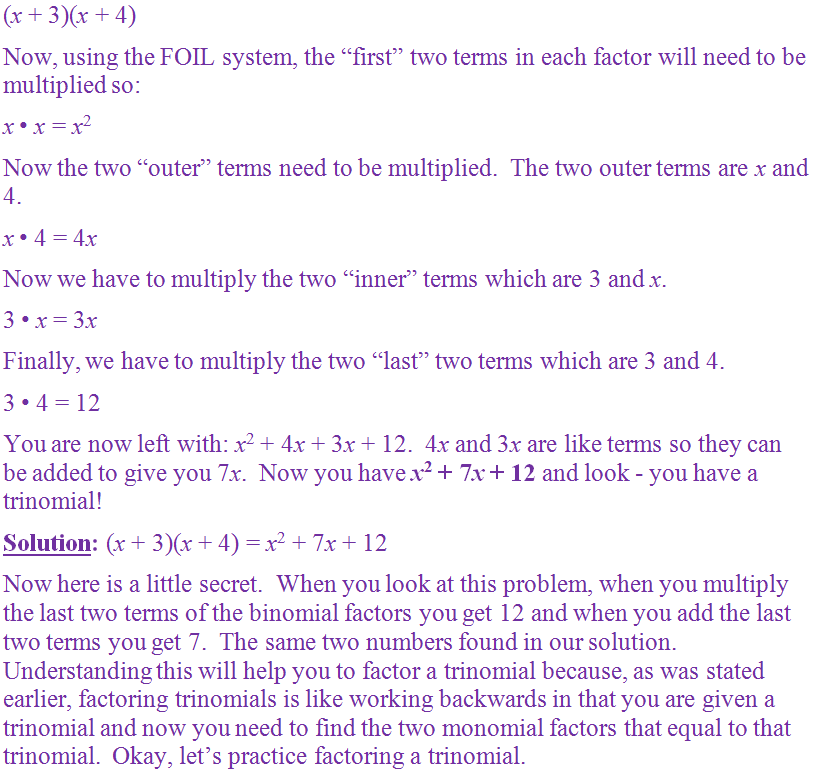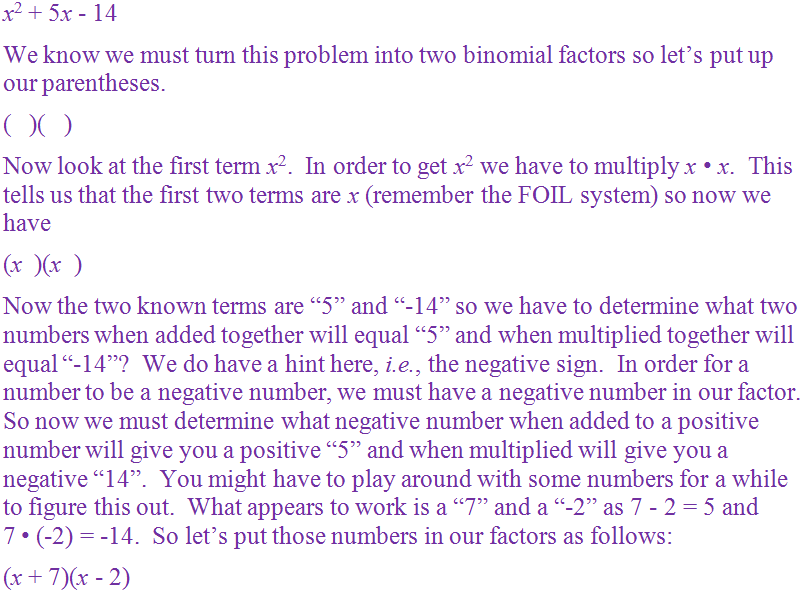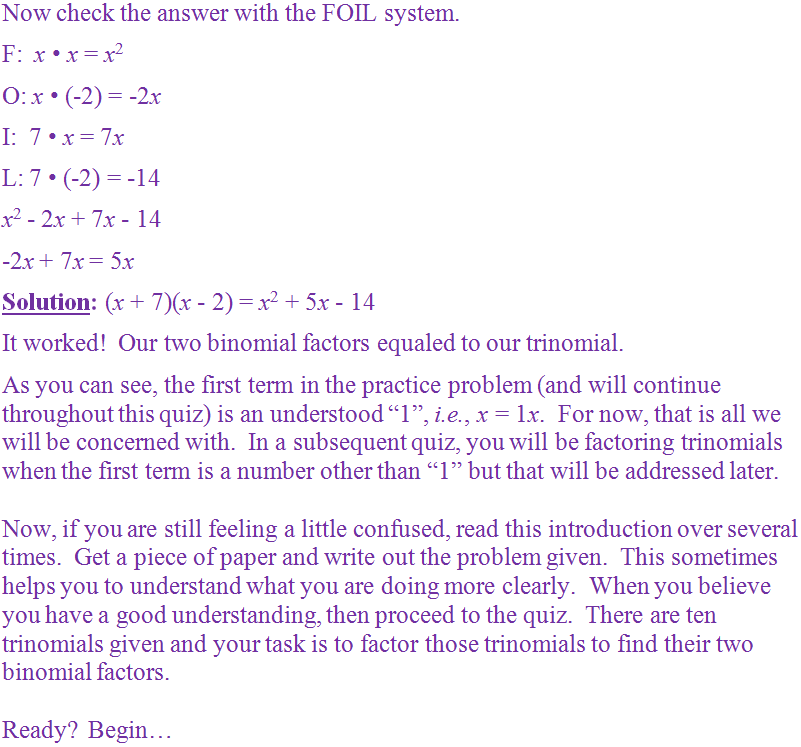1.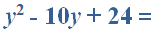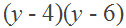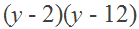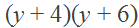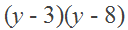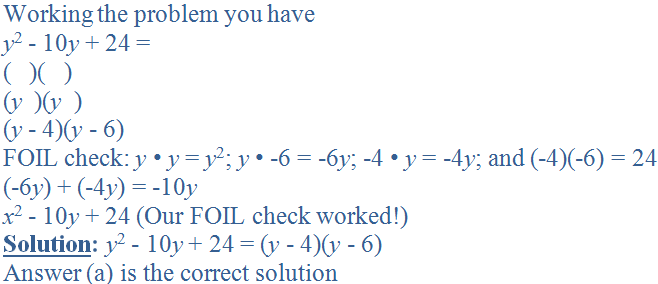2.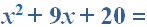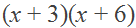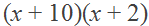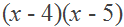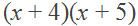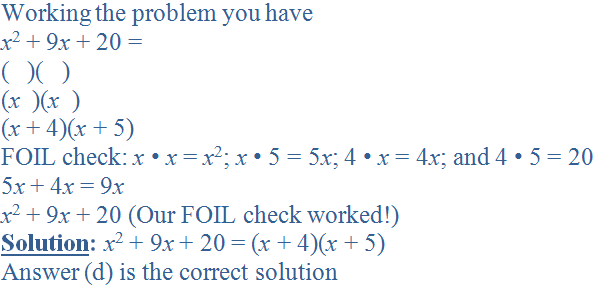3.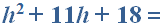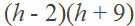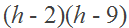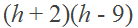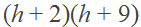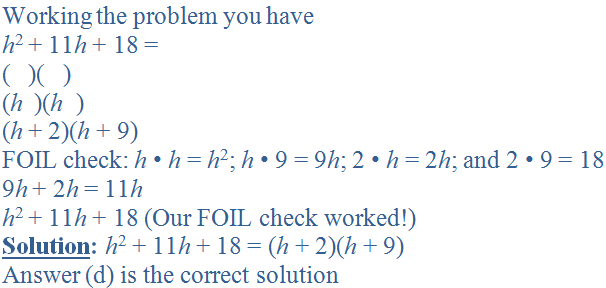4.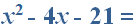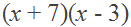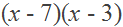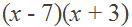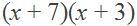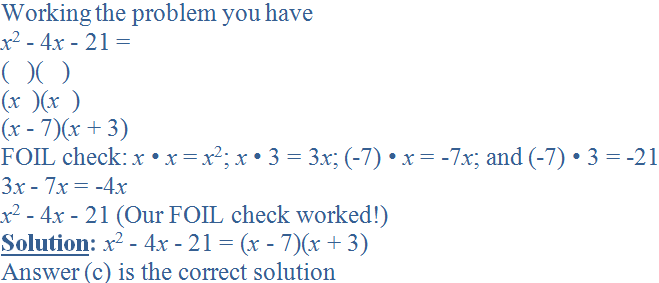5.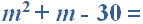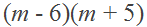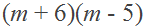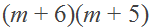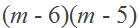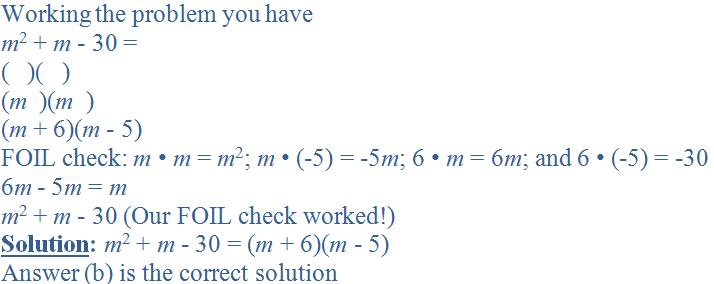6.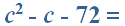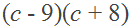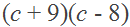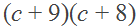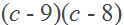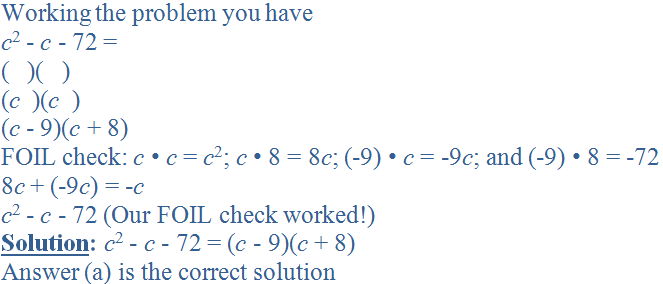7.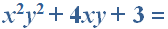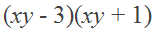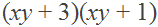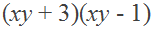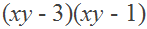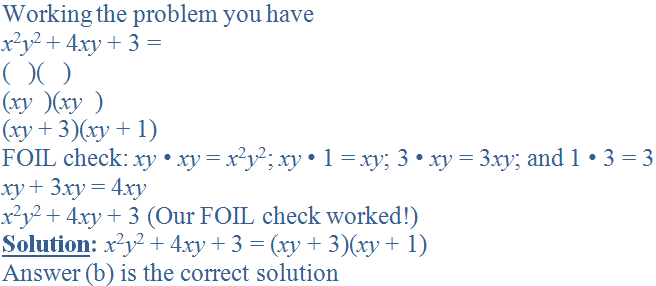8.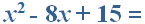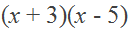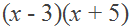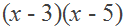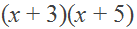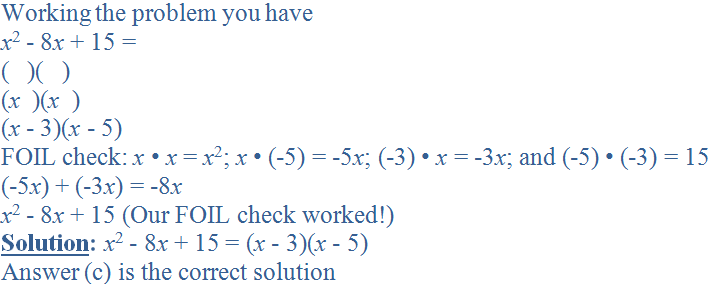9.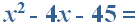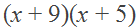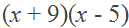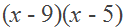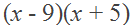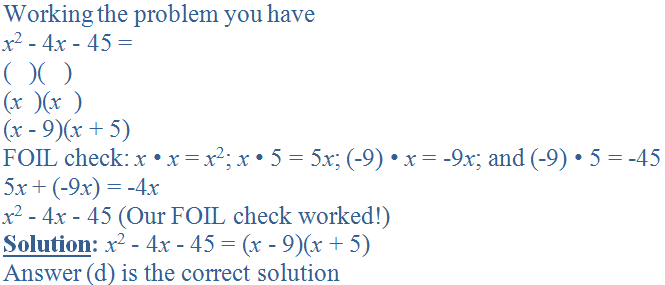10.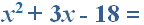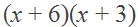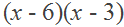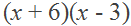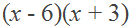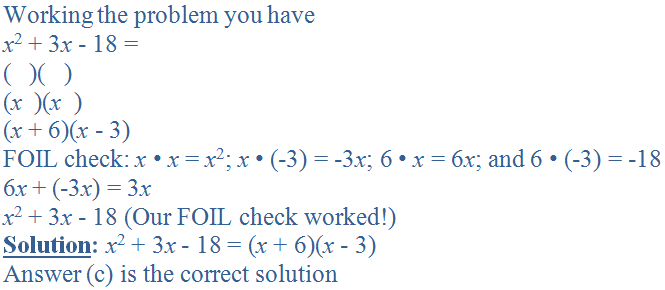Author:  Christine G. Broome